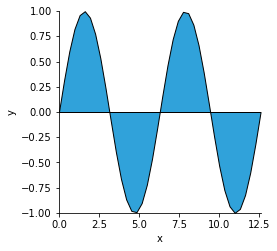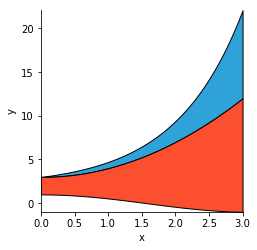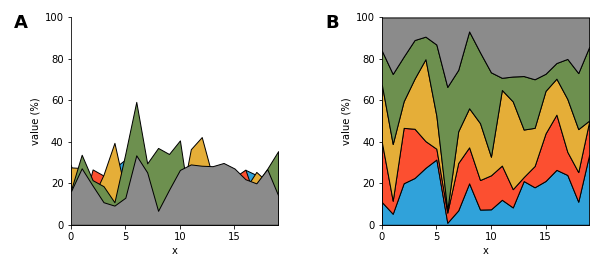# Area¶

Title
Area Element
Dependencies
Matplotlib
Backends
Bokeh
Matplotlib
Plotly
In :
import numpy as np
import holoviews as hv
hv.extension('matplotlib')Area elements are Curve elements where the area below the line is filled. Like Curve elements, Area elements are used to display the development of quantitative values over an interval or time period. Area Elements may also be stacked to display multiple data series in a cumulative fashion over the value dimension.

The data of an Area Element should be tabular with one key dimension representing the samples over the interval or the timeseries and one or two value dimensions. A single value dimension will fill the area between the curve and the x-axis, while two value dimensions will fill the area between the curves. See the Tabular Datasets user guide for supported data formats, which include arrays, pandas dataframes and dictionaries of arrays.

#### Area under the curve¶

By default the Area Element draws just the area under the curve, i.e. the region between the curve and the origin.

In :
xs = np.linspace(0, np.pi*4, 40)
hv.Area((xs, np.sin(xs)))

Out:#### Area between curves¶

When supplied a second value dimension the area is defined as the area between two curves.

In :
X  = np.linspace(0,3,200)
Y = X**2 + 3
Y2 = np.exp(X) + 2
Y3 = np.cos(X)
hv.Area((X, Y, Y2), vdims=['y', 'y2']) * hv.Area((X, Y, Y3), vdims=['y', 'y3'])

Out:#### Stacked areas¶

Areas are also useful to visualize multiple variables changing over time, but in order to be able to compare them the areas need to be stacked. To do this, use the Area.stack classmethod to stack multiple Area elements in an (Nd)Overlay.

In this example we will generate a set of 5 arrays representing percentages and create an Overlay of them. Then we simply call the stack method with this overlay to get a stacked area chart.

In :
values = np.random.rand(5, 20)
percentages = (values/values.sum(axis=0)).T*100

overlay = hv.Overlay([hv.Area(percentages[:, i], vdims=[hv.Dimension('value', unit='%')]) for i in range(5)])
overlay + hv.Area.stack(overlay)

Out:For full documentation and the available style and plot options, use hv.help(hv.Area).

Download this notebook from GitHub (right-click to download).